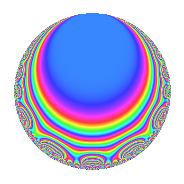# Properties

 Label 122.2.aLevel 122 Weight 2 Character orbit a Rep. character $$\chi_{122}(1,\cdot)$$ Character field $$\Q$$ Dimension 6 Newform subspaces 3 Sturm bound 31 Trace bound 1

# Related objects

## Defining parameters

 Level: $$N$$ = $$122 = 2 \cdot 61$$ Weight: $$k$$ = $$2$$ Character orbit: $$[\chi]$$ = 122.a (trivial) Character field: $$\Q$$ Newform subspaces: $$3$$ Sturm bound: $$31$$ Trace bound: $$1$$ Distinguishing $$T_p$$: $$3$$

## Dimensions

The following table gives the dimensions of various subspaces of $$M_{2}(\Gamma_0(122))$$.

Total New Old
Modular forms 17 6 11
Cusp forms 14 6 8
Eisenstein series 3 0 3

The following table gives the dimensions of the cuspidal new subspaces with specified eigenvalues for the Atkin-Lehner operators and the Fricke involution.

$$2$$$$61$$FrickeDim.
$$+$$$$+$$$$+$$$$1$$
$$+$$$$-$$$$-$$$$2$$
$$-$$$$+$$$$-$$$$3$$
Plus space$$+$$$$1$$
Minus space$$-$$$$5$$

## Trace form

 $$6q - 2q^{3} + 6q^{4} + 2q^{5} + 4q^{7} + 4q^{9} + O(q^{10})$$ $$6q - 2q^{3} + 6q^{4} + 2q^{5} + 4q^{7} + 4q^{9} - 8q^{11} - 2q^{12} + 2q^{13} + 4q^{14} - 16q^{15} + 6q^{16} - 8q^{17} - 2q^{19} + 2q^{20} - 6q^{22} + 4q^{23} - 4q^{25} - 4q^{26} - 8q^{27} + 4q^{28} - 4q^{29} - 12q^{30} - 4q^{31} + 4q^{33} - 4q^{34} - 12q^{35} + 4q^{36} - 8q^{37} - 4q^{38} + 4q^{39} - 8q^{41} - 12q^{42} + 20q^{43} - 8q^{44} + 18q^{45} + 12q^{47} - 2q^{48} + 38q^{49} + 24q^{50} + 8q^{51} + 2q^{52} + 8q^{53} - 24q^{54} - 8q^{55} + 4q^{56} + 24q^{57} + 6q^{58} - 32q^{59} - 16q^{60} - 2q^{61} - 2q^{62} + 16q^{63} + 6q^{64} - 6q^{65} + 16q^{66} + 28q^{67} - 8q^{68} - 44q^{69} - 2q^{70} + 20q^{71} + 20q^{73} + 22q^{74} - 2q^{75} - 2q^{76} + 6q^{77} + 12q^{78} - 8q^{79} + 2q^{80} - 18q^{81} + 16q^{82} - 14q^{83} + 20q^{85} + 4q^{86} - 6q^{88} - 12q^{89} + 16q^{90} + 24q^{91} + 4q^{92} + 20q^{93} - 28q^{94} - 12q^{95} + 14q^{97} - 8q^{98} - 40q^{99} + O(q^{100})$$

## Decomposition of $$S_{2}^{\mathrm{new}}(\Gamma_0(122))$$ into newform subspaces

Label Dim. $$A$$ Field CM Traces A-L signs $q$-expansion
$$a_2$$ $$a_3$$ $$a_5$$ $$a_7$$ 2 61
122.2.a.a $$1$$ $$0.974$$ $$\Q$$ None $$-1$$ $$-2$$ $$1$$ $$-5$$ $$+$$ $$+$$ $$q-q^{2}-2q^{3}+q^{4}+q^{5}+2q^{6}-5q^{7}+\cdots$$
122.2.a.b $$2$$ $$0.974$$ $$\Q(\sqrt{13})$$ None $$-2$$ $$1$$ $$0$$ $$5$$ $$+$$ $$-$$ $$q-q^{2}+(1-\beta )q^{3}+q^{4}+(-1+\beta )q^{6}+\cdots$$
122.2.a.c $$3$$ $$0.974$$ 3.3.229.1 None $$3$$ $$-1$$ $$1$$ $$4$$ $$-$$ $$+$$ $$q+q^{2}+\beta _{2}q^{3}+q^{4}+(-\beta _{1}-\beta _{2})q^{5}+\cdots$$

## Decomposition of $$S_{2}^{\mathrm{old}}(\Gamma_0(122))$$ into lower level spaces

$$S_{2}^{\mathrm{old}}(\Gamma_0(122)) \cong$$ $$S_{2}^{\mathrm{new}}(\Gamma_0(61))$$$$^{\oplus 2}$$